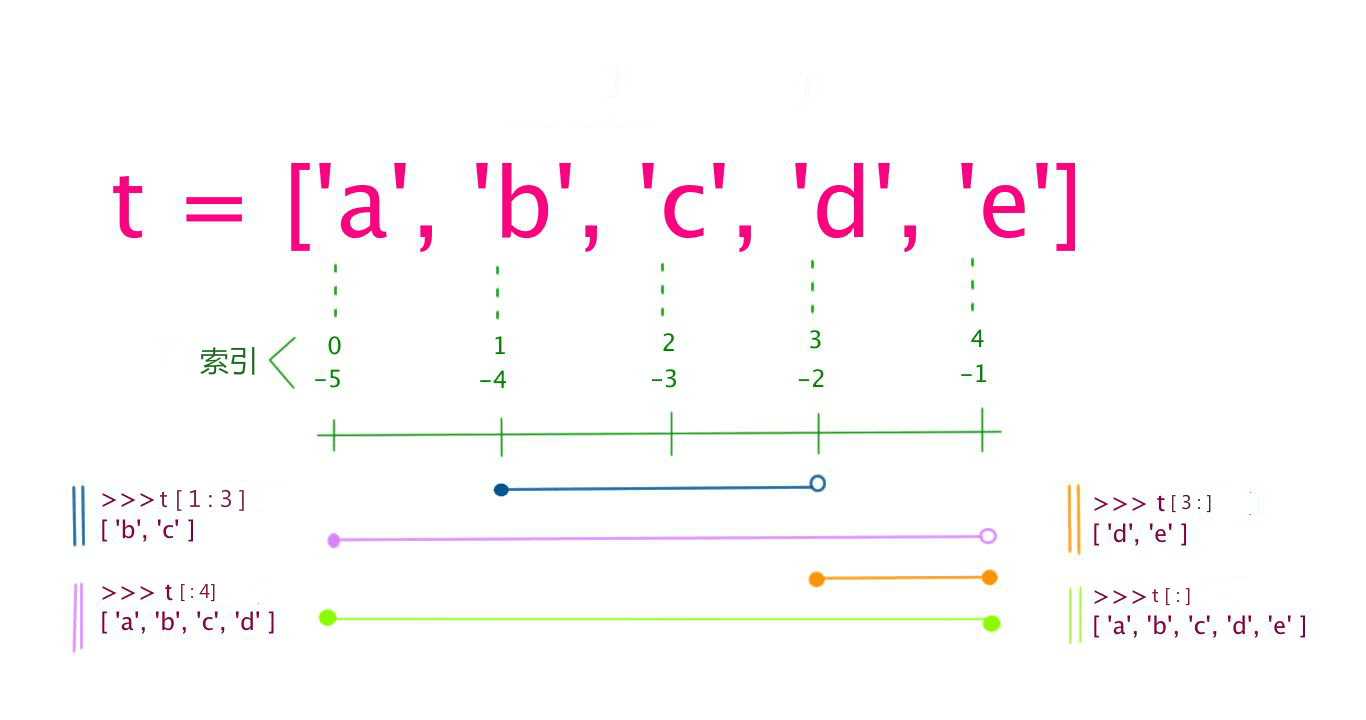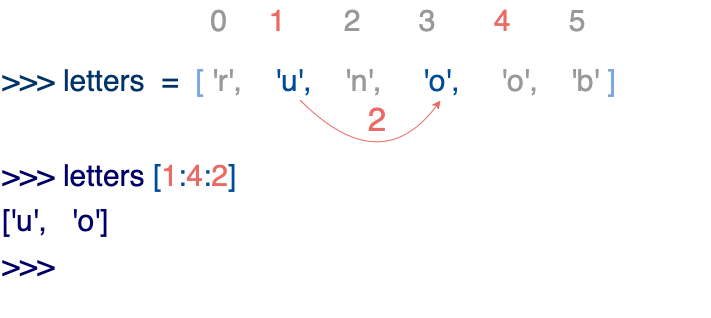# Python3基础之Python3基本数据类型

Python3.X作者：顾小北日期：2021-04-28点击：719

Python 中的变量不需要声明。每个变量在使用前都必须赋值，变量赋值以后该变量才会被创建。

## 实例(Python 3.0+)

#!/usr/bin/python3

counter = 100          # 整型变量
miles   = 1000.0       # 浮点型变量
name    = "runoob"     # 字符串

print (counter)
print (miles)
print (name)

```100
1000.0
runoob
```

### 多个变量赋值

Python允许你同时为多个变量赋值。例如：

```a = b = c = 1
```

`a, b, c = 1, 2, "runoob"`

## 标准数据类型

Python3 中有六个标准的数据类型：

• Number（数字）
• String（字符串）
• List（列表）
• Tuple（元组）
• Set（集合）
• Dictionary（字典）

Python3 的六个标准数据类型中：

• 不可变数据（3 个）：Number（数字）、String（字符串）、Tuple（元组）；
• 可变数据（3 个）：List（列表）、Dictionary（字典）、Set（集合）。

## Number（数字）

Python3 支持 int、float、bool、complex（复数）

```>>> a, b, c, d = 20, 5.5, True, 4+3j
>>> print(type(a), type(b), type(c), type(d))
<class 'int'> <class 'float'> <class 'bool'> <class 'complex'>
```

## 实例

>>> a = 111
>>> isinstance(a, int)
True
>>>

isinstance 和 type 的区别在于：

• type()不会认为子类是一种父类类型。
• isinstance()会认为子类是一种父类类型。
```>>> class A:
...     pass
...
>>> class B(A):
...     pass
...
>>> isinstance(A(), A)
True
>>> type(A()) == A
True
>>> isinstance(B(), A)
True
>>> type(B()) == A
False
```

```var1 = 1
var2 = 10
```

del语句的语法是：

```del var1[,var2[,var3[....,varN]]]
```

```del var
del var_a, var_b```

## 实例

>>> 5 + 4  # 加法
9
>>> 4.3 - 2 # 减法
2.3
>>> 3 * 7  # 乘法
21
>>> 2 / 4  # 除法，得到一个浮点数
0.5
>>> 2 // 4 # 除法，得到一个整数
0
>>> 17 % 3 # 取余
2
>>> 2 ** 5 # 乘方
32

• 1、Python可以同时为多个变量赋值，如a, b = 1, 2。
• 2、一个变量可以通过赋值指向不同类型的对象。
• 3、数值的除法包含两个运算符：/ 返回一个浮点数，// 返回一个整数。
• 4、在混合计算时，Python会把整型转换成为浮点数。

### 数值类型实例

intfloatcomplex
100.03.14j
10015.2045.j
-786-21.99.322e-36j
08032.3e+18.876j
-0490-90.-.6545+0J
-0x260-32.54e1003e+26J
0x6970.2E-124.53e-7j

Python还支持复数，复数由实数部分和虚数部分构成，可以用a + bj,或者complex(a,b)表示， 复数的实部a和虚部b都是浮点型

## String（字符串）

Python中的字符串用单引号 ' 或双引号 " 括起来，同时使用反斜杠 \ 转义特殊字符。

`变量[头下标:尾下标]`## 实例

#!/usr/bin/python3

str = 'Runoob'

print (str)          # 输出字符串
print (str[0:-1])    # 输出第一个到倒数第二个的所有字符
print (str)       # 输出字符串第一个字符
print (str[2:5])     # 输出从第三个开始到第五个的字符
print (str[2:])      # 输出从第三个开始的后的所有字符
print (str * 2)      # 输出字符串两次，也可以写成 print (2 * str)
print (str + "TEST") # 连接字符串

```Runoob
Runoo
R
noo
noob
RunoobRunoob
RunoobTEST
```

Python 使用反斜杠 \ 转义特殊字符，如果你不想让反斜杠发生转义，可以在字符串前面添加一个 r，表示原始字符串：

## 实例

>>> print('Ru\noob')
Ru
oob
>>> print(r'Ru\noob')
Ru\noob
>>>

## 实例

>>> word = 'Python'
>>> print(word, word)
P n
>>> print(word[-1], word[-6])
n P

• 1、反斜杠可以用来转义，使用r可以让反斜杠不发生转义。
• 2、字符串可以用+运算符连接在一起，用*运算符重复。
• 3、Python中的字符串有两种索引方式，从左往右以0开始，从右往左以-1开始。
• 4、Python中的字符串不能改变。

## List（列表）

List（列表） 是 Python 中使用最频繁的数据类型。

`变量[头下标:尾下标]`## 实例

#!/usr/bin/python3

list = [ 'abcd', 786 , 2.23, 'runoob', 70.2 ]
tinylist = [123, 'runoob']

print (list)            # 输出完整列表
print (list)         # 输出列表第一个元素
print (list[1:3])       # 从第二个开始输出到第三个元素
print (list[2:])        # 输出从第三个元素开始的所有元素
print (tinylist * 2)    # 输出两次列表
print (list + tinylist) # 连接列表

```['abcd', 786, 2.23, 'runoob', 70.2]
abcd
[786, 2.23]
[2.23, 'runoob', 70.2]
[123, 'runoob', 123, 'runoob']
['abcd', 786, 2.23, 'runoob', 70.2, 123, 'runoob']
```

## 实例

>>> a = [1, 2, 3, 4, 5, 6]
>>> a = 9
>>> a[2:5] = [13, 14, 15]
>>> a
[9, 2, 13, 14, 15, 6]
>>> a[2:5] = []   # 将对应的元素值设置为 []
>>> a
[9, 2, 6]

List 内置了有很多方法，例如 append()、pop() 等等，这在后面会讲到。

• 1、List写在方括号之间，元素用逗号隔开。
• 2、和字符串一样，list可以被索引和切片。
• 3、List可以使用+操作符进行拼接。
• 4、List中的元素是可以改变的。

Python 列表截取可以接收第三个参数，参数作用是截取的步长，以下实例在索引 1 到索引 4 的位置并设置为步长为 2（间隔一个位置）来截取字符串：## 实例

def reverseWords(input):

# 通过空格将字符串分隔符，把各个单词分隔为列表
inputWords = input.split(" ")

# 翻转字符串
# 假设列表 list = [1,2,3,4],
# list=1, list=2 ，而 -1 表示最后一个元素 list[-1]=4 ( 与 list=4 一样)
# inputWords[-1::-1] 有三个参数
# 第一个参数 -1 表示最后一个元素
# 第二个参数为空，表示移动到列表末尾
# 第三个参数为步长，-1 表示逆向
inputWords=inputWords[-1::-1]

# 重新组合字符串
output = ' '.join(inputWords)

return output

if __name__ == "__main__":
input = 'I like runoob'
rw = reverseWords(input)
print(rw)

`runoob like I`

## 实例

#!/usr/bin/python3

tuple = ( 'abcd', 786 , 2.23, 'runoob', 70.2  )
tinytuple = (123, 'runoob')

print (tuple)             # 输出完整元组
print (tuple)          # 输出元组的第一个元素
print (tuple[1:3])        # 输出从第二个元素开始到第三个元素
print (tuple[2:])         # 输出从第三个元素开始的所有元素
print (tinytuple * 2)     # 输出两次元组
print (tuple + tinytuple) # 连接元组

```('abcd', 786, 2.23, 'runoob', 70.2)
abcd
(786, 2.23)
(2.23, 'runoob', 70.2)
(123, 'runoob', 123, 'runoob')
('abcd', 786, 2.23, 'runoob', 70.2, 123, 'runoob')
```

## 实例

>>> tup = (1, 2, 3, 4, 5, 6)
>>> print(tup)
1
>>> print(tup[1:5])
(2, 3, 4, 5)
>>> tup = 11  # 修改元组元素的操作是非法的
Traceback (most recent call last):
File "<stdin>", line 1, in <module>
TypeError: 'tuple' object does not support item assignment
>>>

```tup1 = ()    # 空元组
tup2 = (20,) # 一个元素，需要在元素后添加逗号
```

string、list 和 tuple 都属于 sequence（序列）。

• 1、与字符串一样，元组的元素不能修改。
• 2、元组也可以被索引和切片，方法一样。
• 3、注意构造包含 0 或 1 个元素的元组的特殊语法规则。
• 4、元组也可以使用+操作符进行拼接。

## Set（集合）

```parame = {value01,value02,...}

set(value)```

## 实例

#!/usr/bin/python3

print(sites)   # 输出集合，重复的元素被自动去掉

# 成员测试
if 'Runoob' in sites :
print('Runoob 在集合中')
else :
print('Runoob 不在集合中')

# set可以进行集合运算
b = set('alacazam')

print(a)

print(a - b)     # a 和 b 的差集

print(a | b)     # a 和 b 的并集

print(a & b)     # a 和 b 的交集

print(a ^ b)     # a 和 b 中不同时存在的元素

```{'Zhihu', 'Baidu', 'Taobao', 'Runoob', 'Google', 'Facebook'}
Runoob 在集合中
{'b', 'c', 'a', 'r', 'd'}
{'r', 'b', 'd'}
{'b', 'c', 'a', 'z', 'm', 'r', 'l', 'd'}
{'c', 'a'}
{'z', 'b', 'm', 'r', 'l', 'd'}
```

## 实例

#!/usr/bin/python3

dict = {}
dict['one'] = "1 - 菜鸟教程"
dict     = "2 - 菜鸟工具"

tinydict = {'name': 'runoob','code':1, 'site': 'www.runoob.com'}

print (dict['one'])       # 输出键为 'one' 的值
print (dict)           # 输出键为 2 的值
print (tinydict)          # 输出完整的字典
print (tinydict.keys())   # 输出所有键
print (tinydict.values()) # 输出所有值

```1 - 菜鸟教程
2 - 菜鸟工具
{'name': 'runoob', 'code': 1, 'site': 'www.runoob.com'}
dict_keys(['name', 'code', 'site'])
dict_values(['runoob', 1, 'www.runoob.com'])
```

## 实例

>>> dict([('Runoob', 1), ('Google', 2), ('Taobao', 3)])
{'Runoob': 1, 'Google': 2, 'Taobao': 3}
>>> {x: x**2 for x in (2, 4, 6)}
{2: 4, 4: 16, 6: 36}
{'Runoob': 1, 'Google': 2, 'Taobao': 3}
>>>

• 1、字典是一种映射类型，它的元素是键值对。
• 2、字典的关键字必须为不可变类型，且不能重复。
• 3、创建空字典使用 { }

## Python数据类型转换

int(x [,base])

float(x)

complex(real [,imag])

str(x)

repr(x)

eval(str)

tuple(s)

list(s)

set(s)

dict(d)

frozenset(s)

chr(x)

ord(x)

hex(x)

oct(x)